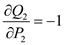# Quiz 14: Pricing Techniques and Analysis

Price elasticity of demand in U.S. is estimated to be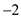and price elasticity of demand is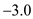overseas markets. Overseas market is more elastic as comparison to the domestic market. Marginal cost is \$40. Following is the condition for equilibrium:Elasticity of demand in overseas market is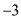. Marginal cost is \$15 in overseas market. Following is the equilibrium price in the overseas market:It has been proved that highly elastic demand reduces the price of output. Domestic price is higher in comparison to overseas for high elasticity of demand in overseas market.
Price elasticity of demand for first class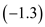Price elasticity of demand for unrestricted coach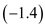Price elasticity of demand for restricted discount coach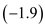Following is the condition for equilibrium:Calculate the values of prices by equating the marginal revenue function with the marginal cost:a) Total Profit function Demand function for two classes of goods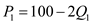Total Revenue for manufactured itemTotal Revenue for semi-manufactured item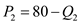Total Cost function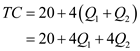Total Profit Function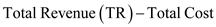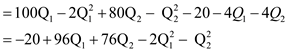b) Profit maximizing price and output Differentiating the total profit function with respect to the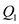:-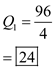Differentiating the total profit function with respect to the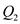:-Substituting the value of output in inverse demand functionc) Marginal Revenue in each market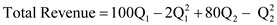Substituting the values of outputMarginal Cost for manufactured good is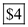Substituting the values of outputMarginal Cost for semi-manufactured good isd) Profit if different prices are charged in markets Total Profit Function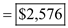e) Profit if same price is charged Price of both manufacture and semi-manufactured items should be equal. Or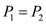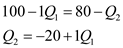Total Profit Function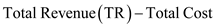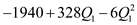Differentiating it with respect toEquation the marginal profit with zeroSubstituting the value of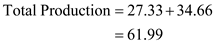Following is the price of manufactured item:-Profit at equalized pricef) Difference in profit level between differential pricing and uniform pricing Elasticity of demand manufactured good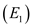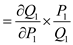Differentiating with respect to the price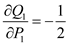Elasticity of demand manufactured good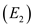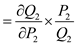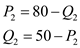Differentiating with respect to the price